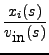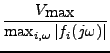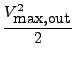Next: Wide-band signal Up: Maximum Signal Level Previous: Maximum Signal Level   Contents

#### Sinusoid with unknown frequency

A very popular assumption is that the input signal is a sinusoid with unknown frequency. In the time domain:

 Vin(t) = VAsin(ωt). (12)
Let vin(s) be the Laplace transform of Vin(t) . If the transfer function in the Laplace domain from the input of the filter to the output of integrator i is fi :

 fi(s) =, (13)
the amplitude of the signal at the output of this integrator will be

 | xi(jω)| = | fi(jω)| VA (14)
Let the maximum output signal amplitude at the output of any integrator be Vmax . The maximum filter input amplitude Vmax, in is then:

 Vmax, in =(15)
and the maximum filter output signal level is

 Vmax, out = Vmax, in(| H(jω)|). (16)
The mean-squared maximal output level is=(17)

FA will use this assumption to calculate the maximum output signal level and the dynamic range if the scale_inf flag is set by

set: scale_inf
This is the default.Next: Wide-band signal Up: Maximum Signal Level Previous: Maximum Signal Level   Contents
2009-06-03